# MySQL索引解析（联合索引/最左前缀/覆盖索引/索引下推）

### 2. 索引类型

1. 哈希表
2. 有序数组
3. 搜索树（重点）

#### 2.3 B+树索引（InnoDB）

``````CREATE TABLE T(
id int primary key,
k int not null,
index (k)
)engine=InnoDB;
``````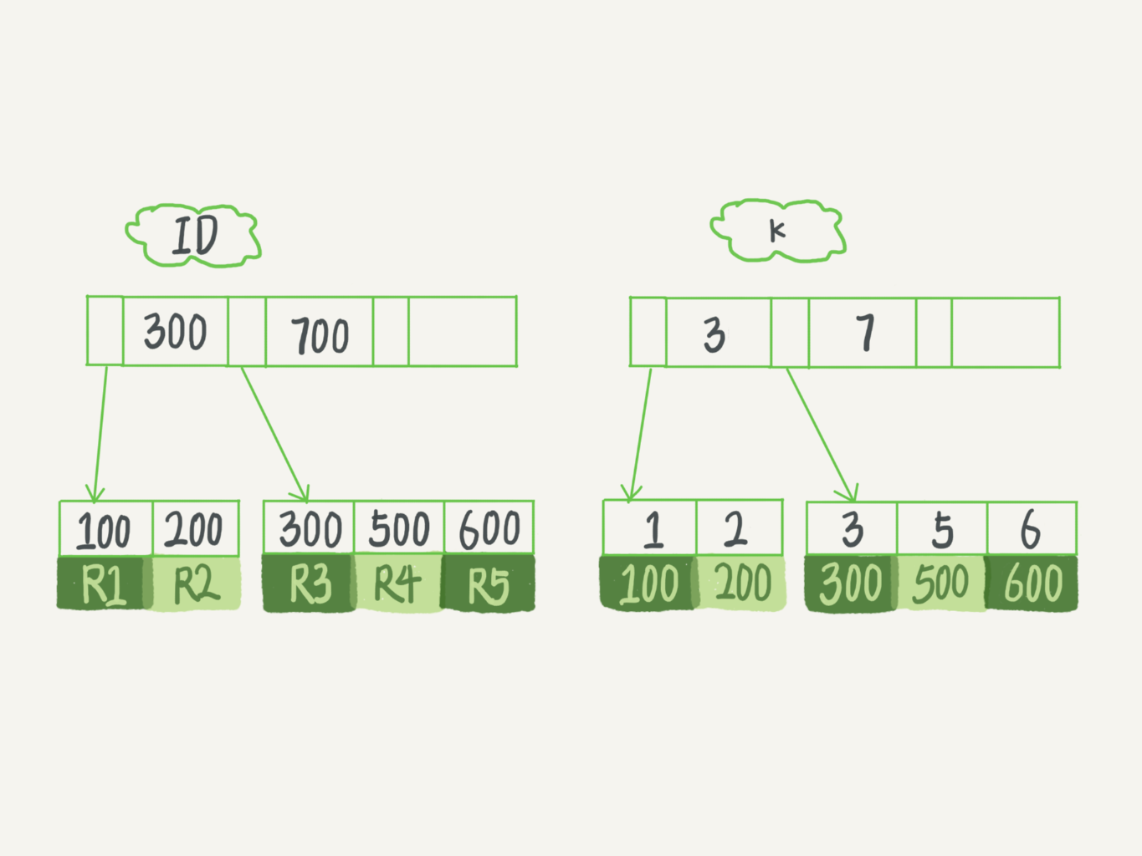• 主键索引的叶子结点存的是整条记录，主键索引也被称为聚簇索引（clustered index）
• 非主键索引的叶子结点存的是主键的值，非主键索引也被称为二级索引（secondary index）/普通索引/辅助索引

• 如果语句是 select * from T where ID=500，即主键查询，则只需要搜索ID这棵树。
• 如果语句是 select * from T where k=5，即非主键索引查询，则需要先搜索k索引树，得到ID的值为500，再到ID索引树搜索一次。从非主键索引回到主键索引的过程称为回表

### 3. 联合索引

``````// 表
userid int not null,
)ENGINE=InnoDB;

// 插入数据

// 添加索引
// （或用key关键字也一样的）
``````

``````select * from buylog where userid=2;
``````

``````select * from buylog where userid=1 order by buy_date desc limit 2;
``````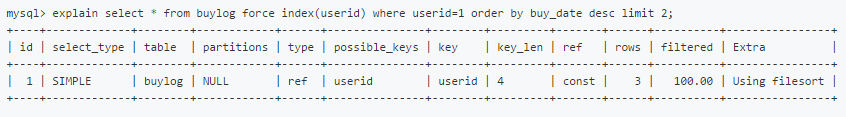``````select ... from xxx where a=xxx;
select ... from xxx where a=xxx order by b;
``````

``````select ... from xxx where b=xxx;
``````

``````select ... from xxx where a=xxx order by b;
select ... from xxx where a=xxx and b=xxx order by c;
``````

``````select ... from xxx where a=xxx order by c;
``````

### 4. 最左前缀原则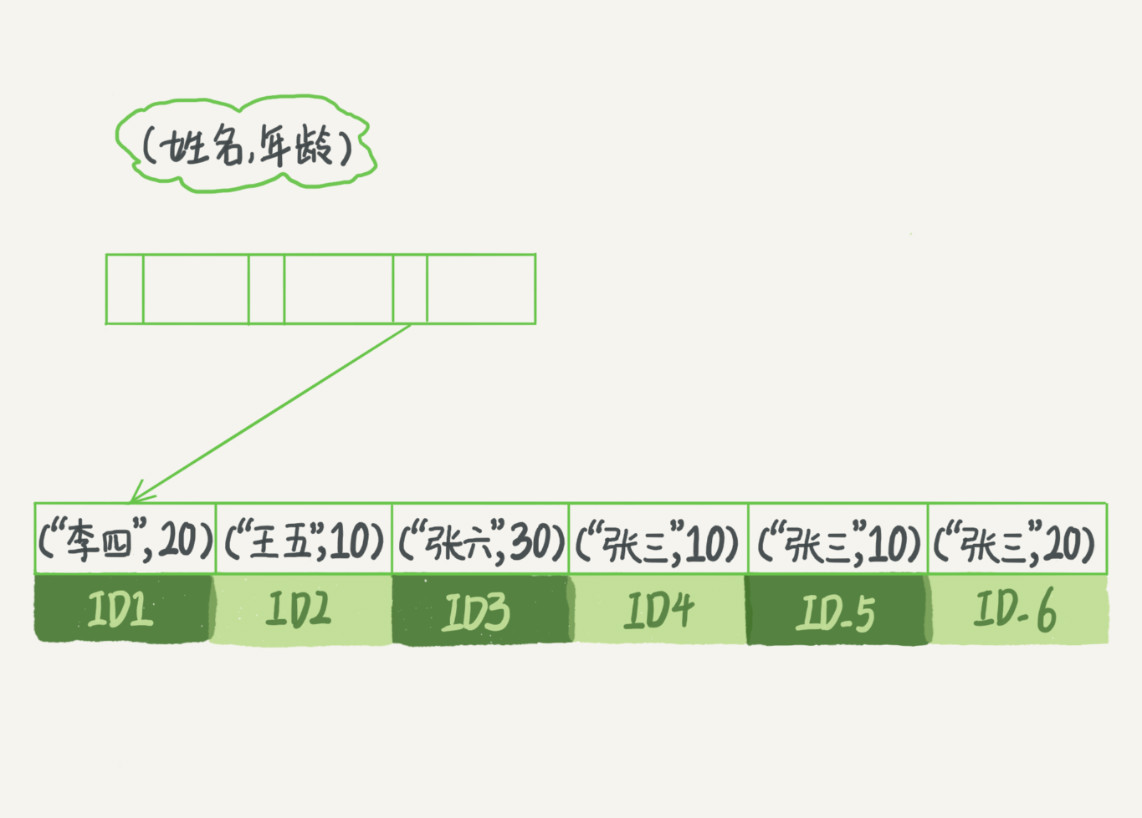### 5. 覆盖索引

``````select * from T where k between 3 and 5;
``````

1. 在 k 索引树上找到 k=3 的记录，取得 ID = 300；
2. 再到 ID 索引树查到 ID=300 对应的 R3；
3. 在 k 索引树取下一个值 k=5，取得 ID=500；
4. 再回到 ID 索引树查到 ID=500 对应的 R4；
5. 在 k 索引树取下一个值k=6，不满足条件，循环结束。

``````select ID from T where k between 3 and 5;
``````

``````select count(*) from T;
``````

### 6. 索引下推

``````mysq> select * from tuser where name like '张 %' and age=10 and ismale=1;
``````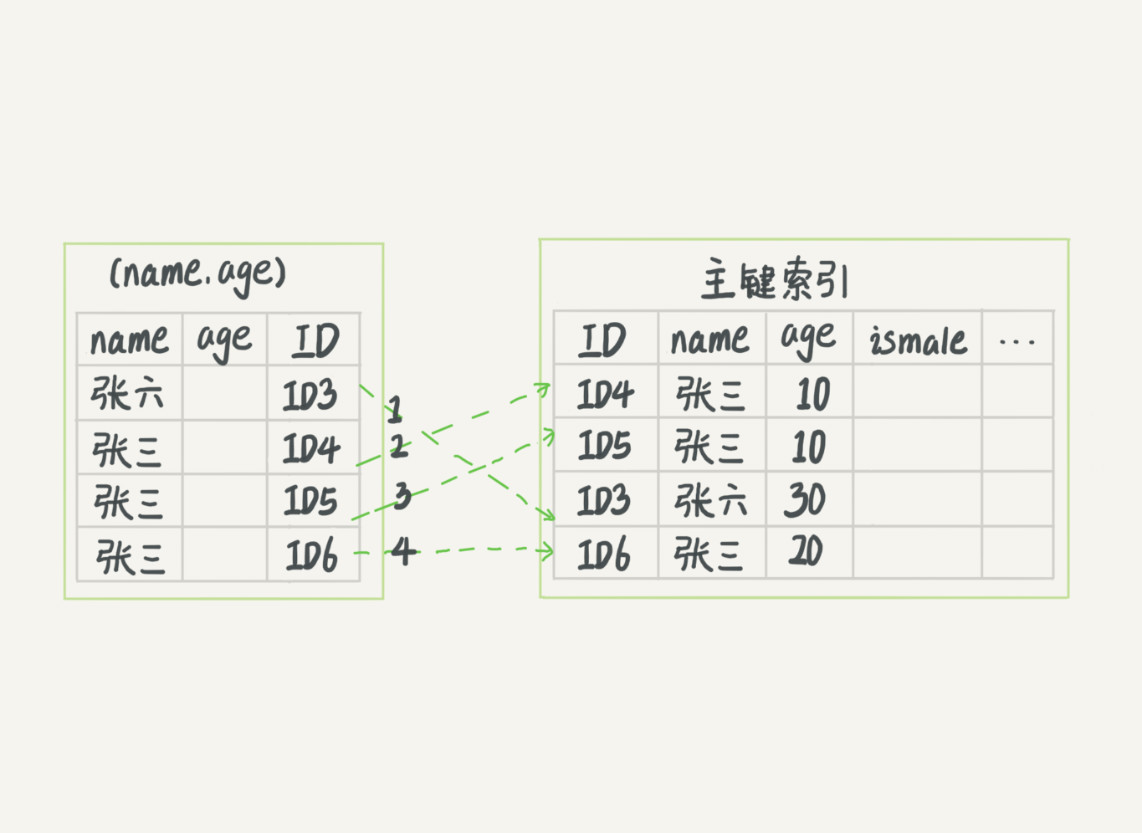（图1）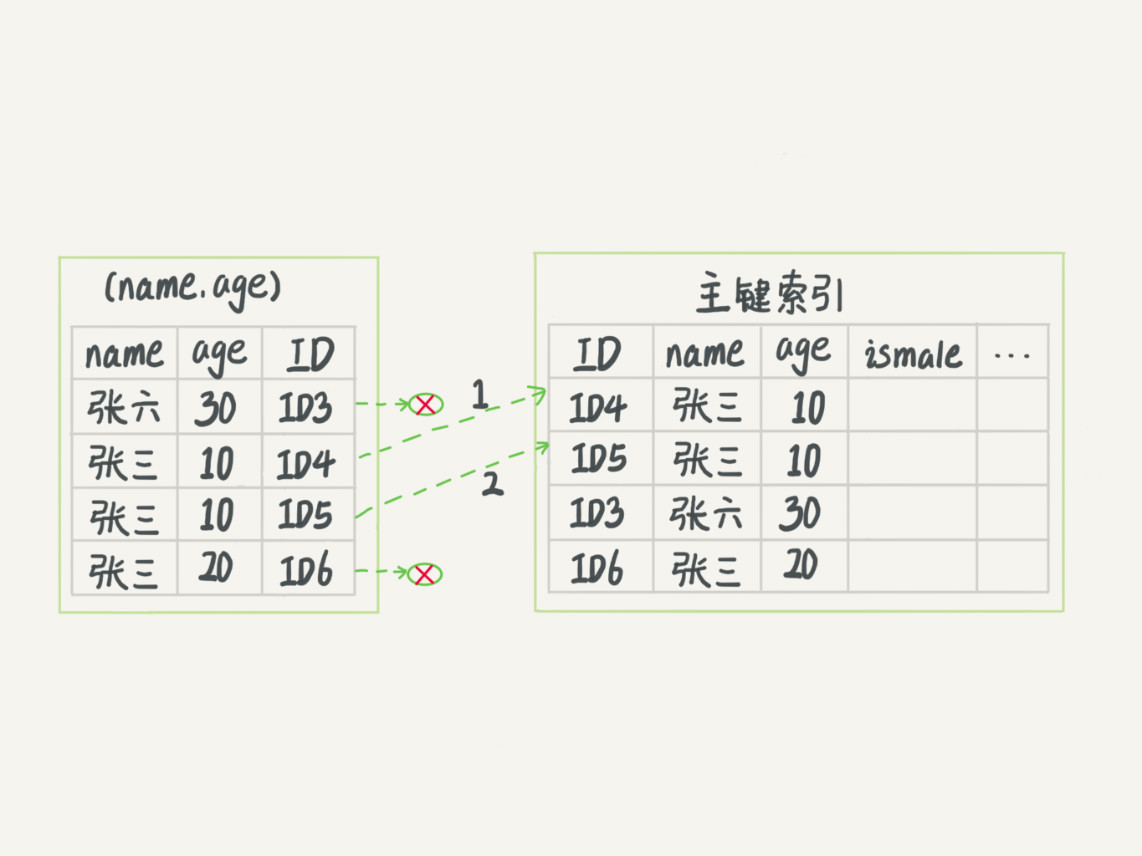（图2）

### 总结：

• 索引的常见数据结构有哪些？（哈希表，有序数组，B+树），它们分别有怎样的特点？分别适合哪些应用场景？
• 主键索引（也称聚簇索引）和非主键索引（也称辅助索引/二级索引）的概念是什么？回表的概念又是什么？
• 什么是联合索引，什么是最左前缀原则？（由于MySQL的最左前缀特性，建立联合索引的时候对字段的顺序应该要多考虑）。什么是覆盖索引？能否清楚的说出Index Condition Pushdown优化的原理？### 觉得文章有用就打赏一下文章作者

#### 支付宝扫一扫打赏#### 微信扫一扫打赏• 回顶